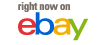# Casio AL-8S

The Casio AL-8S is a handheld calculator from 1976. It measures 82mm x 140mm x 20mm and weighs 130g without batteries. The calculator is powered by two AA batteries or an optional AC adapter.

The AL-8S has a slab-style plastic case in dark brown and white. The eight digit blue vacuum fluorescent display is recessed and tilted. Above the display is a metallic sticker with the Casio name. The keyboard panel is aluminum with blue labels.

This four function calculator includes additional features like square root, percentages, fractions, date calculations, remainder function, memory, and a total key. The decimal point location can be switched between floating and fixed.

The calculator uses a Hitachi HD36133 processor from July 1976. Other components include a single tube eight digit VFD, diodes, capacitors, resistors, and transistors. The main board connects to the keyboard with copper wires. A small voltage booster circuit is present.

Some logic quirks:

• The C key clears the last digit entered, while AC clears the entire calculator
• Overflow is suppressed – entering a 9th digit is ignored
• Negative numbers show a minus sign taking the leftmost digit spot
• Overflow and divide by zero lock up the calculator in an unrecoverable state
• Constant second number is available for all four functions
• Memory storage is not indicated
• Fraction calculations are possible but limited
• Date calculations allow finding the day of the week
• The remainder key shows the remainder and quotient from division
• Grand total adds all results sequentially

• Overflow on number input is suppressed – inputting a ninth digit is ignored
• Negative numbers are shown with a “-” to the immediate left of a number but as there is no ninth digit to travel into, you are limited to seven digit negative numbers.
• An overflow shows the result with no indication of error except that it hooks up and is not recoverable.
• Divide by zero results in “E” in the far right (first) digit and is not recoverable
• There is selectable constant on all four functions by double hitting the operator; i.e. (3)(X)(X)(2)(=) gives “6”, (5)(=) gives “15” and so on.
• There is no indication of memory store – you have to remember it!
• This calculator suffers the negative zero bug. Try (1)(-)(2)(=) to give “-1” then add one with (+)(1)(=) to give “-0”
• The fraction function works by inputting using the (a b/c) key: to enter 1 1⁄2 key in (1) (a b/c)(1) (a b/c)(2) (a b/c) and the display will show the result with reverse lower “L” signs. You can add subtract, multiply and divide with fractions but you will lose the fractional display if your answer becomes irrational or has a numerator/denominator greater than three digits. You can convert the fraction to a decimal by using the (rem) key
• The date function can be used to find the day; enter 5 Feb 1961 by keying in (6)(1)(date)(2)(date)(5)(date) and the display shows “61.02.05-0” the last zero indicating it is a Sunday. You can subtract another date to find how many days between the two.
• A switch allows you to select Floating [F], and two-fixed decimal mode with round down [CUT] or round [5/4] options.
• The (rem) key is a remainder function. Key in (6)(/)(4)(rem) and you will get “1” and (rem) again will show the remainder “2”
• The (GT) key is a grand total; this will show the accumulated sum of every result of the (=) key.

The Casio AL-8S is a mid-1970s calculator with typical Casio quality but limited logic and error recovery. It includes useful features like fractions and date calculations but oddities like the negative zero bug. This model represents an early stage in Casio’s calculator evolution.

#####As an eBay associate I earn a small commission on qualifying purchases which helps support this site.